# Technitrain Courses In London This Autumn

I’ve just put up a bunch of new courses (including a dedicated Power Query course!) on the Technitrain site that will be running this autumn in London. They are:

I hope to see some of you there! Don’t forget you can also get 10% off on my MDX training videos and lots of other great MS BI content at Project Botticelli using the discount code TECHNITRAIN2014.

# Using List.Generate() To Make Multiple Replacements Of Words In Text In Power Query

Recently I had a request for help from someone who wanted to do the following in Power Query: take a piece of text and then, using a table, search for all of the occurrences of the words in one column of the table in the text and replace those words with those in the other column. So, for example, given these two tables in Excel:You want to take the table on the left and for each piece of text replace the words in the ‘Word To Replace’ column of the right-hand table with those in the ‘Replace With’ column of the right-hand table. The output would therefore be:An interesting challenge in itself, and one I solved first of all using a recursive function. Here’s some code showing how I did it:

`let`

`    //Get table of word replacements`

`    Replacements = Excel.CurrentWorkbook(){[Name="Replacements"]}[Content],`

`    //Get table containing text to change`

`    TextToChange = Excel.CurrentWorkbook(){[Name="Text"]}[Content],`

`    //Get a list of all words to replace`

`    WordsToReplace = Table.Column(Replacements, "Word To Replace"),`

`    //Get a list of all words to replace with`

`    WordsToReplaceWith = Table.Column(Replacements, "Replace With"),`

`    //Recursive function to do the replacement`

`    ReplacementFunction = (InputText, Position)=> `

`    let `

`     //Use Text.Replace to do each replace`

`     ReplaceText = Text.Replace(`

`            InputText, `

`            WordsToReplace{Position}, `

`            WordsToReplaceWith{Position})`

`    in`

`     //If we have reached the end of the list of replacements`

`     if Position=List.Count(WordsToReplace)-1 `

`      then `

`      //return the output of the query`

`      ReplaceText `

`      else `

`      //call the function again`

`      @ReplacementFunction(ReplaceText, Position+1),`

`    //Add a calculated column to call the function on every row in the table`

`    //containing text to change`

`    Output = Table.AddColumn(TextToChange, "Changed Text", each ReplacementFunction([Text], 0))`

`    `

`in`

`    Output`

It does the job, but… after thinking about this some more, I wondered if there was a better way. A lot of my recent Power Query blog posts have used recursive functions, but are they a Good Thing? So I asked on the forum, and as usual the nice people on the Power Query dev team answered very promptly (that’s one of the things I like about the Power Query dev team – they engage with their users). Recursive functions are indeed something that should be avoided if there is an alternative, and in this case List.Generate() can be used instead. Here’s how:

`let`

`    //Get table of word replacements`

`    Replacements = Excel.CurrentWorkbook(){[Name="Replacements"]}[Content],`

`    //Get table containing text to change`

`    TextToChange = Excel.CurrentWorkbook(){[Name="Text"]}[Content],`

`    //Get list of words to replace`

`    WordsToReplace = Table.Column(Replacements, "Word To Replace"),`

`    //Get list of words to replace them with`

`    WordsToReplaceWith = Table.Column(Replacements, "Replace With"),`

`    //A non-recursive function to do the replacements`

`    ReplacementFunction = (InputText)=> `

`     let`

`       //Use List.Generate() to do the replacements`

`       DoReplacement = List.Generate(`

`                          ()=> [Counter=0, MyText=InputText], `

`                          each [Counter]<=List.Count(WordsToReplaceWith), `

`                          each [Counter=[Counter]+1, `

`                                MyText=Text.Replace(`

`                                         [MyText], `

`                                         WordsToReplace{[Counter]}, `

`                                         WordsToReplaceWith{[Counter]})], `

`                          each [MyText]),`

`       //Return the last item in the list that`

`       //List.Generate() returns`

`       GetLastValue = List.Last(DoReplacement)`

`     in`

`      GetLastValue,`

`    //Add a calculated column to call the function on every row in the table`

`    //containing the text to change`

`    Output = Table.AddColumn(TextToChange, "Changed Text", each ReplacementFunction([Text]))`

`in`

`    Output`

List.Generate() is a very powerful function indeed, albeit one that took me a while to understand properly. It’s a bit like a FOR loop even if it’s a function that returns a list. Here’s what each of the parameters I’m passing to the function in the example above do:

•  ()=> [Counter=0, MyText=InputText] returns a function that itself returns a record (a record is a bit like a table with just one row in it). The record contains two fields: Counter, which has the value 0, and MyText which is given the value of the text where the values are to be replaced. This record is the initial value that List.Generate() will modify at each iteration.
• each [Counter]<=List.Count(WordsToReplaceWith) returns a function too. An each expression is a quick way of declaring a function that takes one, unnamed parameter, and in this case the value that will be passed to this parameter is a record of the same structure as the one declared in the previous bullet. The expression [Counter] gets the value of the Counter field from that record. The function returns a boolean value, true when the value in the [Counter] field of the record is less than or equal to the number of items in the list of words to replace. List.Generate() returns a list, and while this function returns true it will keep on iterating and adding new items to the list it returns.
• each [Counter=[Counter]+1, MyText=Text.Replace([MyText], WordsToReplace{[Counter]}, WordsToReplaceWith{[Counter]})] returns yet another function, once again declared using an each expression. The function here takes the record from the current iteration and returns the record to be used at the next iteration: a record where the value of the Counter field is increased by one, and where the value of the MyText field has one word replaced. The word that gets replaced in MyText is the word in the (zero-based) row number given by Counter in the ‘Word To Replace’ column; this word is replaced by the word in the row number given by Counter in the ‘Replace With’ column.
• each [MyText] returns a very simple function, one that returns the value from the MyText field of the record from the current iteration. It’s the value that this function returns that is added to the list returned by List.Generate() at every iteration.

To illustrate this, here’s a simplified example showing how List.Generate() works in this case:

`let`

`    WordsToReplace = {"cat", "dog", "mat"},`

`    WordsToReplaceWith = {"fish", "snake", "ground"},`

`    Demo = List.Generate(`

`                          ()=> [Counter=0, MyText="the cat and the dog sat on the mat"], `

`                          each [Counter]<=List.Count(WordsToReplaceWith), `

`                          each [Counter=[Counter]+1, `

`                                MyText=Text.Replace(`

`                                         [MyText], `

`                                         WordsToReplace{[Counter]}, `

`                                         WordsToReplaceWith{[Counter]})], `

`                          each [MyText])`

` `

`in`

`    Demo`

The output of this query is the list: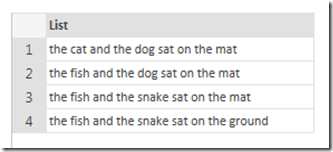This list can be written as (with the words changed at each iteration highlighted):

{“the cat and the dog sat on the mat”, “the fish and the dog sat on the mat”,  “the fish and the snake sat on the mat”, “the fish and the snake sat on the ground”}

So, another useful function to know about. I’m slowly getting to grips with all this functional programming!

# Using Slicer Selections In The CubeSet Function

I had an interesting challenge from a customer yesterday – one of those problems that I’d known about for a long time but never got round to working out the solution for…

Consider the following PivotTable, based on a PowerPivot model using Adventure Works data, in Excel 2010: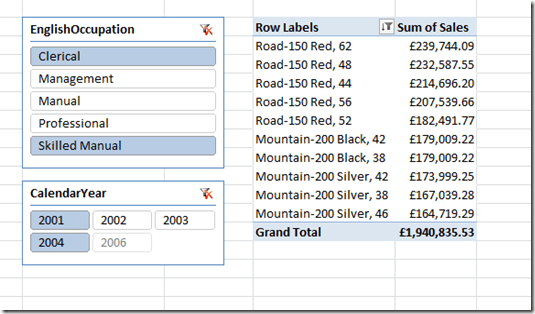It shows the top 10 products by the measure Sum of Sales. There are two slicers, and the top 10 shown in the PivotTable reflects the selections made in the slicers. All of this works fine. But what if you want to use Excel cube functions to do the same thing? You can write the MDX for the top 10 products quite easily and use it in the CubeSet() function in your worksheet, but how can you get your MDX set expression to respect the selection made in the slicers?

The solution to this problem is very similar to the trick I showed here – finding the selected items in a slicer is not easy! Here are the steps I followed to do it:

The bad news about this technique is that it doesn’t work in Excel 2013 and Power Pivot. It’s no longer possible to create MDX calculated measures on Power Pivot models in Excel 2013, alas. It will work if you’re using any version of Excel from 2007 on against Analysis Services and, as I show here, Excel 2010 and PowerPivot. If you are using Power Pivot and Excel 2013 it might be possible to create a DAX measure to do the same as the MDX I’ve used here (I’m wondering if the technique Jason describes here will work). It would certainly be possible to use CubeRankedMember() to find each item selected in the slicer, as Erik Svensen shows here, and then use Excel formulas to find the MDX unique name for each selected member and concatenate these unique names to create the set expression that my calculated measures return, but that’s a topic for another post. This really should be a lot easier than it is…

# SSAS Multidimensional Formula Engine Caching and Locale-Dependent Properties

One subject I have blogged about many times here is how the use of certain MDX functions and features prevents the Formula Engine from caching the result of a calculation for longer than the lifetime of a query (see here and here for just two examples). Reading the new SSAS 2012/2014 Performance Guide, I spotted the following:

The use of MDX functions that are locale-dependent (such as CAPTION or .Properties) prevents the use of the global cache, because different sessions may be connected with different locales and cached results for one locale may not be correct for another locale.

It’s a bit vague but here’s an example of it happening. Take the following calculated measure for the Adventure Works cube:

`CREATE MEMBER CURRENTCUBE.MEASURES.TEST AS`

`IIF(`

`[Date].[Date].CURRENTMEMBER.PROPERTIES("MEMBER_NAME")="July 1, 2001",`

`[Measures].[Internet Sales Amount],`

`[Measures].[Internet Tax Amount]);`

If you run the following query, first on a cold cache and then on a warm cache:

`select`

`{measures.test} `

`on 0,`

`[Date].[Date].[Date].members`

`on 1`

`from`

`[Adventure Works]`

In Profiler you will see that the second time it is run, the values for the calculated measure are returning from the Formula Engine cache: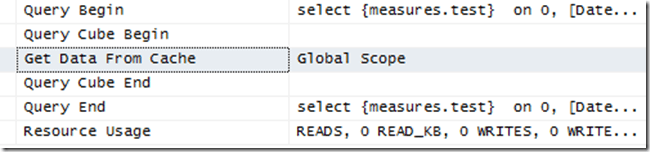Here, the Get Data From Cache event is showing that on the second execution of the query the Formula Engine cache is being used.

However, if you change the calculation so it uses the MEMBER_CAPTION property instead of the MEMBER_NAME property, like so:

`CREATE MEMBER CURRENTCUBE.MEASURES.TEST AS`

`IIF(`

`[Date].[Date].CURRENTMEMBER.PROPERTIES("MEMBER_CAPTION")="July 1, 2001",`

`[Measures].[Internet Sales Amount],`

`[Measures].[Internet Tax Amount]);`

Then run the query again, on a cold cache then a warm cache, you will see the following: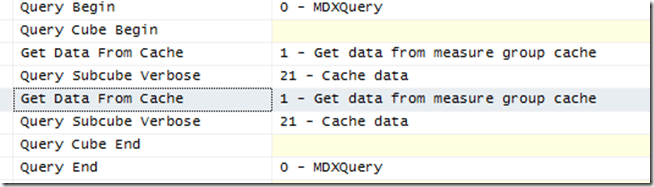Here the Storage Engine cache is being used – and since the query only contains a single calculated measure, this means that the Formula Engine cache is not being used, and may result in noticeably worse overall query performance if your calculation is expensive.

The reason there is a difference between MEMBER_NAME and MEMBER_CAPTION is that the former property will return the same value for all users, but the latter may return different values for users in different locales. Therefore it is not safe to cache the results of calculations that use the MEMBER_CAPTION property because these calculations could return different values for different users for the same query.

# Calculating Ages In MDX

One area where I see people have a lot of problems when they’re learning MDX is when they are working with dates. SQL has a lot of great functionality for working with dates while MDX, to be honest, does not – therefore people tie themselves in knots writing complex MDX expressions (which often don’t perform all that well) to recreate what they would do in SQL. However, as is often the case with MDX, approaching the questions from a completely different angle yields much better results.

For example, let’s imagine that you wanted to calculate the age in years of any customer on any given date. Using the Adventure Works cube you have a Date dimension, which will be how you want to select the date that the age is to be calculated at; there is also, on the Customer dimension, an attribute called Birth Date that gives you each customer’s date of birth. You need a calculated measure that calculates the age of each customer for any date selected on the Date dimension.

If you think about this as a SQL date problem, you’ll probably want to use functions like DateDiff() – one of the VBA functions that is available in MDX (but is not native MDX). The following article shows how DateDiff() and other VBA functions can be used to calculate ages in VBA code:
http://msdn.microsoft.com/en-us/library/aa227466(v=vs.60).aspx

Here’s a query showing how this calculation can be implemented in MDX:

`with`

`--calculate the difference between the years of the `

`--current date and the customer's birth date`

`member measures.yeardiff as`

`DateDiff("yyyy",`

`[Customer].[Customer].currentmember.properties("Birth Date", typed),`

`[Date].[Date].currentmember.member_value`

`)`

`--calculate a value which is the month number`

`--multiplied by 100 plus the day number of the month`

`--for the current date`

`member measures.datemonthday as`

`(month([Date].[Date].currentmember.member_value) * 100)`

`+`

`day([Date].[Date].currentmember.member_value)`

`--calculate a value which is the month number`

`--multiplied by 100 plus the day number of the month`

`--for the customer birth date`

`member measures.birthmonthday as`

`(month([Customer].[Customer].currentmember.properties("Birth Date", typed)) * 100)`

`+`

`day([Customer].[Customer].currentmember.properties("Birth Date", typed))`

` `

`--calculate customer age as the difference in years`

`--minus 1 if the customer's birthday this year is`

`--after the current date`

`member measures.customerage as`

`measures.yeardiff -`

`iif(measures.datemonthday>=measures.birthmonthday,0,1)`

` `

`select`

`--return all dates in 2002 on columns`

`descendants(`

`[Date].[Calendar].[Calendar Year].&,`

`[Date].[Calendar].[Date])`

`on 0,`

`--return all customers in Coff's Harbour on rows`

`descendants(`

`[Customer].[Customer Geography].[City].&[Coffs Harbour]&[NSW],`

`[Customer].[Customer Geography].[Customer])`

`on 1`

`from`

`[Adventure Works]`

`where(measures.customerage)`

The output of the query is this:On my laptop this query, which returns 365 columns and 106 rows, returns in around 3.5 seconds. A few things to point out:

• I’m using the .Member_Value function instead of the .MemberValue function to get the typed date value associated with each date, as Bill Anton’s recent blog post points out that it is marginally faster.
• I’m using .Properties(“Birth Date”, typed) to get the typed date value for each customer’s birth date. If I set the ValueColumn property on the Customer attribute of the Customer dimension, or enabled the hierarchy on the Birth Date attribute, I would be able to use .Member_Value here too and get a very minor performance improvement.
• Most of time spent by the query is taken by the yeardiff calculated member, which uses the DateDiff() function. This isn’t surprising because calling any VBA function in MDX is expensive and something to be avoided.

Performance here is ok, but we can do better. The most important thing to do when tuning any MDX calculation is to try to pre-calculate as much as possible. In this case there are two calculations we can move out of MDX into the structure of the cube:

• The DateDiff() function here just calculates the difference between the years of the two dates. Therefore if you create a new attribute on the Customer dimension to hold the integer representing the year of the customer’s birth date, and use the existing Calendar Year attribute on the Date dimension, you can avoid using DateDiff() completely and do a simple subtraction.
• Likewise, rather than calculating the combination of month and date in the MDX, these values can be pre-calculated in the dimension and then accessed as properties.

Here’s the rewritten version of the calculation, assuming that the following new attributes have been added to the Customer and Date dimensions:

• [Customer].[Birth Year] holds the year of the customer’s birth as an integer
• [Customer].[Birth Month Day] is an integer containing the month number multiplied by 100 plus the day number of the month for the customer’s birth date. For example the date 5th June would have the value 605.
• [Date].[Month Day] is the same as [Customer].[Birth Month Day] but for the Date dimension.
`with`

`member measures.yeardiff as`

`[Date].[Calendar Year].currentmember.member_value`

`-`

`[Customer].[Birth Year].currentmember.member_value`

` `

`member measures.customerage as`

`measures.yeardiff`

`-`

`iif(`

`[Date].[Month Day].currentmember.member_value`

`>=`

`[Customer].[Birth Month Day].currentmember.member_value`

`,0,1)`

` `

`select`

`descendants(`

`[Date].[Calendar].[Calendar Year].&,`

`[Date].[Calendar].[Date])`

`on 0,`

`descendants(`

`[Customer].[Customer Geography].[City].&[Coffs Harbour]&[NSW],`

`[Customer].[Customer Geography].[Customer])`

`on 1`

`from`

`[Adventure Works]`

`where(measures.customerage)`

This query runs in around 1.2 seconds, a significant improvement. Of course if you were to create a completely new fact table that contained the age of every customer on every date in the Date dimension then you wouldn’t need to use any calculated measures and performance would be even better, but that may not be practical if you have a lot of customers and a lot of dates.

# Join Conditions in Power Query, Part 2: Events-In-Progress, Performance and Query Folding

In my last post you saw how to join two tables together using conditions other than the built-in inner, outer and anti join conditions. However, as I mentioned, you need to be aware of some of the performance implications of using this technique – and that is the subject I’ll be looking at in this post.

Let’s take the events-in-progress problem, one that I have blogged about many times in the past (see here, here, here and here for example) and see how we can solve it in Power Query. It’s a very common problem that you encounter when you have a fact table where each row represents an event, there are columns containing the start and end dates of each event, and you want to count the number of events that were in progress on any given date.

Using the Adventure Works DW database, my normal way of illustrating the problem is this: taking the DimDate and FactInternetSales tables, for each date show the number of purchases that had been ordered but not shipped on that date. One way of solving this problem (but not the only way, as my previous blog posts show) is to do a cross join between the DimDate and FactInternetSales tables, then filter the result so that you get the rows where the value in the DateKey column from the DimDate table is between the values in the OrderDateKey and the ShipDateKey columns from the FactInternetSales table.

As a first attempt you might come up with a query like this one, which uses a custom column containing a table value that is subsequently expanded to do a cross join (the technique shown in my previous post):

`let`

`    //Connect to SQL Server`

`    Source = Sql.Database("localhost", "Adventure Works DW"),`

`    //Get data from the DimDate table`

`    dbo_DimDate = Source{[Schema="dbo",Item="DimDate"]}[Data],`

`    //Remove all columns except the DateKey column`

`    RemovedOtherColumns = Table.SelectColumns(dbo_DimDate,{"DateKey"}),`

`    //Insert a custom column that contains the whole of FactInternetSales as a table in each row`

`    InsertedCustom = Table.AddColumn(RemovedOtherColumns, "FactInternetSalesTable", `

`                        each Source{[Schema="dbo",Item="FactInternetSales"]}[Data]),`

`    //Expand this new column to show the OrderDateKey and ShipDateKey columns`

`    #"Expand FactInternetSalesTable" = Table.ExpandTableColumn(InsertedCustom, `

`                        "FactInternetSalesTable", `

`                        {"OrderDateKey", "ShipDateKey"}, `

`                        {"FactInternetSalesTable.OrderDateKey", `

`                        "FactInternetSalesTable.ShipDateKey"}),`

`    //Filter where DateKey is greater than or equal to OrderDateKey and `

`    //DateKey is less than or equal to ShipDateKey`

`    FilteredRows = Table.SelectRows(#"Expand FactInternetSalesTable", `

`                        each [DateKey] >= [FactInternetSalesTable.OrderDateKey] and `

`                        [DateKey] <= [FactInternetSalesTable.ShipDateKey]),`

`    //Find the count of the number of rows grouped by DateKey`

`    GroupedRows = Table.Group(FilteredRows, {"DateKey"}, `

`                        {{"Count", each Table.RowCount(_), type number}})`

`in`

`    GroupedRows`

There is, however, a big problem with this query: on my laptop it runs and runs forever – well, maybe not forever but I cancelled it after several minutes. Some tuning is necessary.

I don’t think anyone outside the Power Query dev team has much experience of performance tuning Power Query yet. However there is one golden rule that I do know: where possible, allow Power Query to push as much of the work back to the data source. This behaviour is known as “query folding” and it’s something that I’ve blogged about, as have Matt Masson and Darren Gosbell. Looking in SQL Server Profiler for the query above it is clear that no query folding is taking place: the only activity visible is Power Query reading the data from the DimDate and FactInternetSales tables separately.

After a bit of trial and error I came up with the following alternative:

`let`

`    //Connect to SQL Server`

`    Source = Sql.Database("localhost", "adventure works dw"),`

`    //Get data from the DimDate table`

`    dbo_DimDate = Source{[Schema="dbo",Item="DimDate"]}[Data],`

`    //Remove all columns except DateKey`

`    RemovedOtherColumns = Table.SelectColumns(dbo_DimDate,{"DateKey"}),`

`    //Add a custom column to DimDate containing the value 1`

`    InsertedCustom = Table.AddColumn(RemovedOtherColumns, "Dummy", each 1),`

`    //Get data from the FactInternetSales table`

`    dbo_FactInternetSales = Source{[Schema="dbo",Item="FactInternetSales"]}[Data],`

`    //Remove all columns except OrderDateKey and ShipDateKey`

`    RemovedOtherColumns1 = Table.SelectColumns(dbo_FactInternetSales,`

`                        {"OrderDateKey", "ShipDateKey"}),`

`    //Add a custom column to FactInternetSales containing the value 1`

`    InsertedCustom1 = Table.AddColumn(RemovedOtherColumns1, "Dummy", each 1),`

`    //Join DimDate and FactInternetSales on the two columns that contain 1`

`    Custom1 = Table.Join(InsertedCustom1, "Dummy", InsertedCustom, "Dummy"),`

`    //Filter rows where DateKey is between OrderDateKey and ShipDateKey`

`    FilteredRows = Table.SelectRows(Custom1, `

`                    each [DateKey] >= [OrderDateKey] and `

`                    [DateKey] <= [ShipDateKey]),`

`    //Group by DateKey and find the number of rows for each date`

`    GroupedRows = Table.Group(FilteredRows, {"DateKey"}, `

`                    {{"Count", each Table.RowCount(_), type number}}),`

`    //Sort dates in ascending order`

`    SortedRows = Table.Sort(GroupedRows,{{"DateKey", Order.Ascending}})`

`in`

`    SortedRows`

This returns the correct result more or less instantly:The main difference between this query and the previous one is how I’m getting a cross join between the two tables. This time I’m creating custom columns on DimDate and FactInternetSales that both contain the value 1, and then doing an inner join between this two tables on the new columns – which of course results in the equivalent of a cross join.

In Profiler I can see the following SQL query being generated by Power Query:

`select [_].[DateKey],`

`    [_].[Count]`

`from `

`(`

`    select [rows].[DateKey] as [DateKey],`

`        count(1) as [Count]`

`    from `

`    (`

`        select [_].[OrderDateKey],`

`            [_].[ShipDateKey],`

`            [_].[Dummy],`

`            [_].[DateKey]`

`        from `

`        (`

`            select [\$Outer].[OrderDateKey],`

`                [\$Outer].[ShipDateKey],`

`                [\$Inner].[Dummy],`

`                [\$Inner].[DateKey]`

`            from `

`            (`

`                select [_].[OrderDateKey] as [OrderDateKey],`

`                    [_].[ShipDateKey] as [ShipDateKey],`

`                    1 as [Dummy]`

`                from `

`                (`

`                    select [OrderDateKey],`

`                        [ShipDateKey]`

`                    from [dbo].[FactInternetSales] as [\$Table]`

`                ) as [_]`

`            ) as [\$Outer]`

`            inner join `

`            (`

`                select [_].[DateKey] as [DateKey],`

`                    1 as [Dummy]`

`                from `

`                (`

`                    select [DateKey]`

`                    from [dbo].[DimDate] as [\$Table]`

`                ) as [_]`

`            ) as [\$Inner] on ([\$Outer].[Dummy] = [\$Inner].[Dummy])`

`        ) as [_]`

`        where [_].[DateKey] >= [_].[OrderDateKey] and [_].[DateKey] <= [_].[ShipDateKey]`

`    ) as [rows]`

`    group by [DateKey]`

`) as [_]`

`order by [_].[DateKey]`

Query folding is definitely taking place now!

The last question to ask here is whether the first query was slow because query folding was not taking place, or slow because of the way the query was written. You can test this quite easily by rewriting the second query to prevent query folding taking place using the Table.Buffer() function. For example, in the second query the step to get the data from the DimDate table is:

dbo_DimDate = Source{[Schema="dbo",Item="DimDate"]}[Data],

To prevent query folding it needs to be altered to:

dbo_DimDate = Table.Buffer(Source{[Schema="dbo",Item="DimDate"]}[Data]),

(The step to get data from the FactInternetSales table needs to be altered in the same way.)

With this change made the query now executes in around a minute. So clearly the new query is more efficient when it is executed inside the Power Query engine itself, without query folding, but the Power Query engine is still nowhere near as fast as SQL Server and query folding gives the best possible performance.

# Join Conditions In Power Query, Part 1

In last week’s post I showed how to create a simple LIKE function in Power Query which could be used in a calculated column. This week I’m going to show you how you can use this function in a condition when joining two tables together.

Consider the following two tables in an Excel worksheet:Let’s say you want to join the Patterns table to the Phrases table, but only return the rows for each pattern where the Like() function returns true. Power Query has good support for different types of joins in the Table.Join() and Table.NestedJoin() functions but it isn’t immediately obvious how to handle join conditions such as this one.

Here’s the M code for a query that shows you how to do this:

`let`

`    //Load Patterns table`

`    PatternsTable = Excel.CurrentWorkbook(){[Name="Patterns"]}[Content],`

`    //Load Phrases table`

`    PhrasesTable = Excel.CurrentWorkbook(){[Name="Phrases"]}[Content],`

`    //Add custom column to Patterns that returns the Phrases table for each row`

`    InsertedCustom = Table.AddColumn(PatternsTable, "AllPhrases", each PhrasesTable),`

`    //Expand the new column`

`    ExpandedTable = Table.ExpandTableColumn(InsertedCustom, "AllPhrases", {"Phrases"}, {"Phrases"}),`

`    //Filter the expanded table using the Like() function`

`    Custom1 = Table.SelectRows(ExpandedTable, each Like([Phrases],[Patterns]))`

`in`

`    Custom1`

What this query does is the following:

• Gets the data from both the Patterns table and the Phrases table. I’ve done this as two steps in the same query for simplicity; you might want to create two separate queries to do this.
• On the Patterns table, add a new custom column that returns the entire Phrases table as a value. The Insert Custom Column dialog and its output will look like this: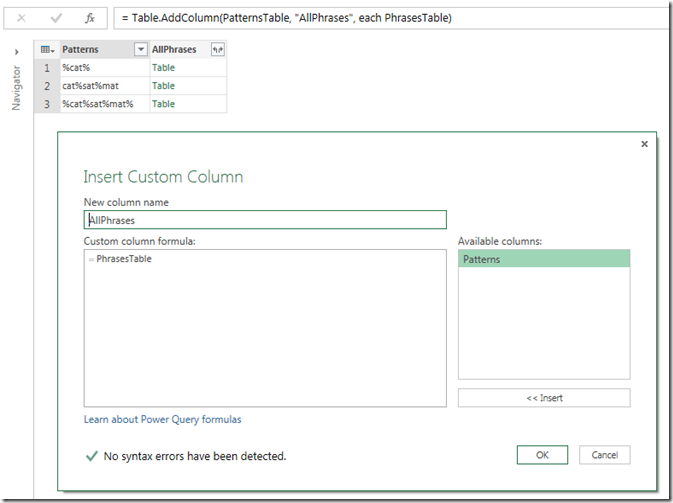• Expands the new custom column so that every row of the Phrases table is displayed against every row of the Patterns table – in effect, it does a cross join between the two tables. Interestingly there is no option to do a cross join using the Table.Join() and Table.NestedJoin() functions.• Finally, it uses the Like() function in Table.SelectRows() as follows:

Table.SelectRows(ExpandedTable, each Like([Phrases],[Patterns]))

…to filter the table from the previous step so that you only get the rows back where the Like() function returns true and the pattern matches the phrase:So… we have a solution to our problem (and you can download the example workbook here). However, be warned: for large tables this approach may not perform well! In part two I’ll show you another example of a complex join condition and show you how different approaches can yield very different performance.